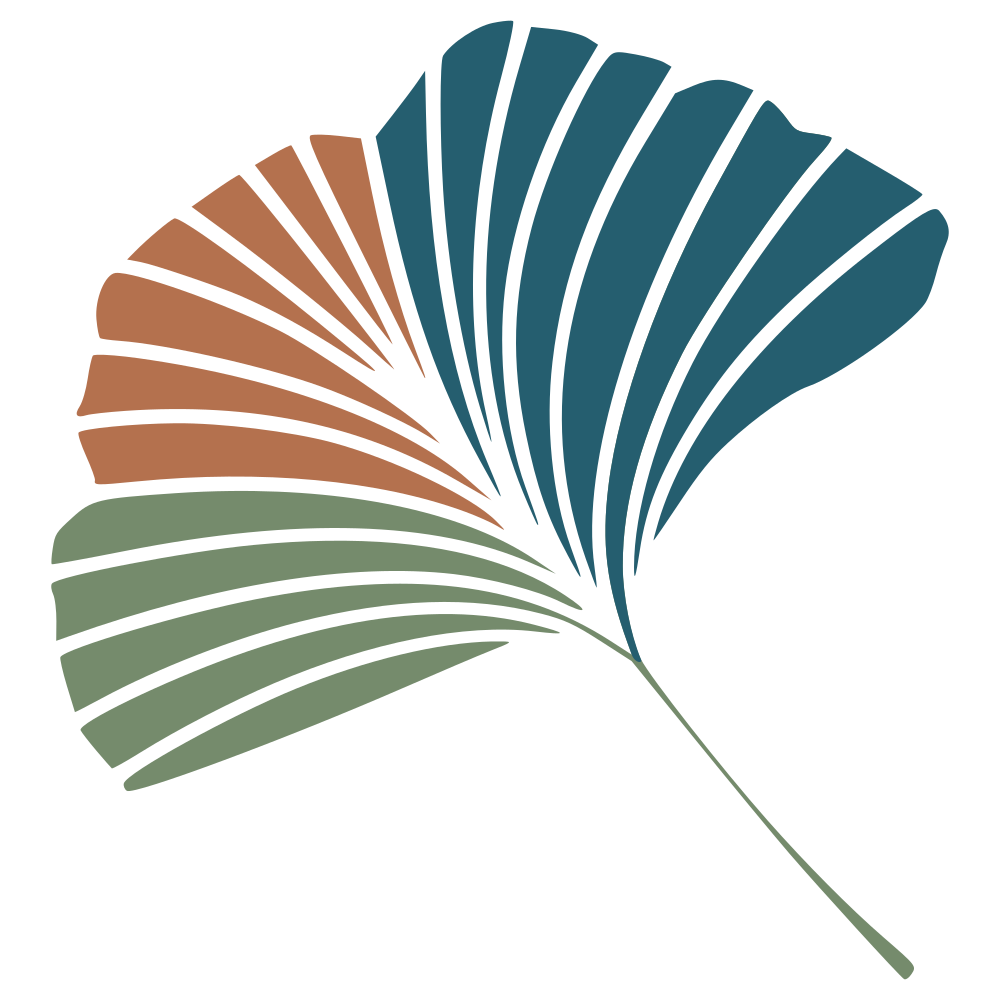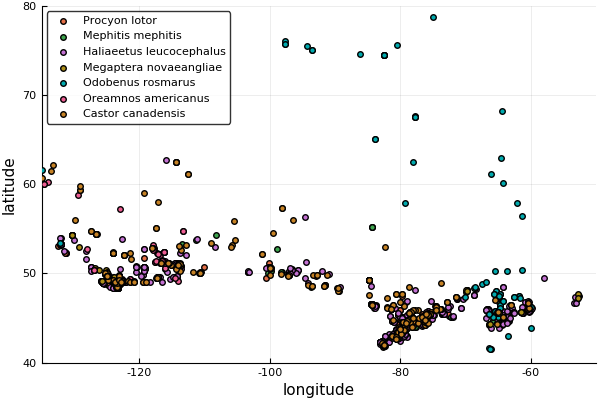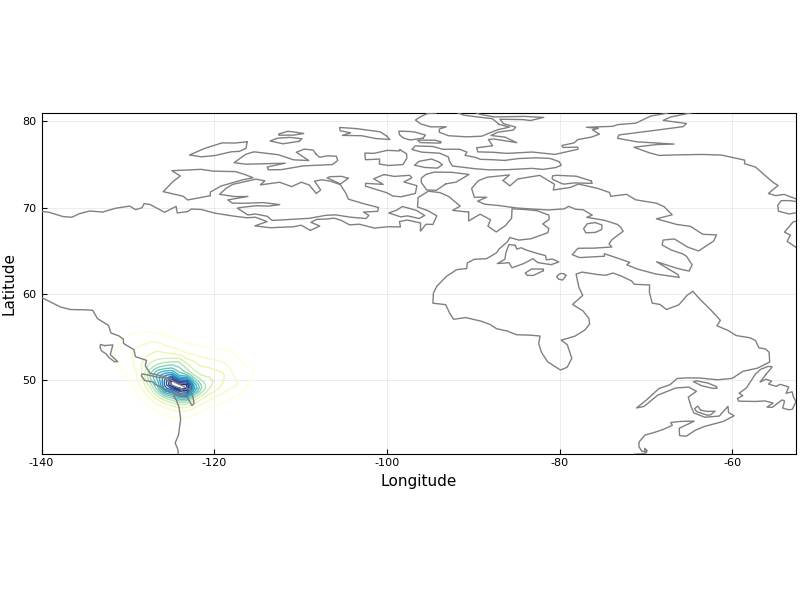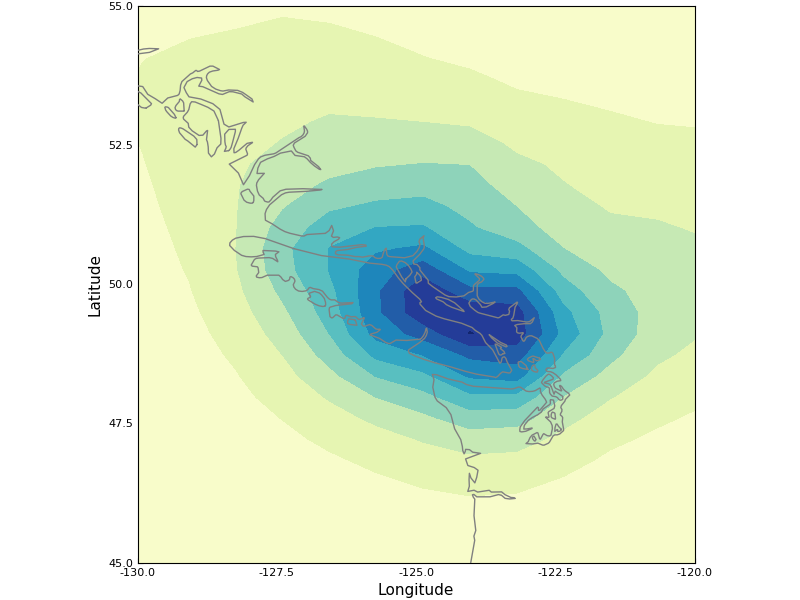# A biogeographic study of Paw Patrol

You guys know about Paw Patrol? It’s a kid’s TV show where a band of pups solve mysteries, help people, and do engage in other kid-friendly antics. It’s pretty great. And a few weeks ago, our son started asking where the pups live. Being dedicated watchers of the show, we replied “Adventure Bay”. But he wanted to know where adventure bay is. Let’s use the power of biogeography to find out.

What we know, is that the Paw Patrol interacts with all sorts of animals. There’s a few mountain goats, beavers, a bald eagle, racoons, skunks, etc. This is enough to narrow down possible locations to North America. Seeing that the show creators are Canadian, then Canada also seems like a safe bet. So we can formulate our question as “where in Canada is it most likely to observe the set of all species appearing in Paw Patrol?”.

A few months ago, I started working on a Julia wrapper on the GBIF API, which albeit not complete, is functional enough to do this. Let’s download it, and load a few other required packages:

Pkg.clone("https://github.com/EcoJulia/GBIF.jl")
using GBIF
using DataFrames
using Query
using StatPlots
using Plot


Once this is done, we need to decide on a list of species – after consulting with our son (and double checking some of them as a family research project), we decided on the following:

species_list = [
"Oreamnos americanus", # Mountain goat
"Haliaeetus leucocephalus", # Bald eagle
"Megaptera novaeangliae", # Humpback whale
"Odobenus rosmarus", # Walrus
"Procyon lotor", # Raccoon
"Mephitis mephitis" # Skunk
];


We can now retrieve their identifier on GBIF:

species_info = Dict{String, Integer}()
for s in species_list
info = species(s, rank=:SPECIES)
species_info[s] = info["speciesKey"]
end


This will create a dictionary, with the species name as the key, and the unique identifier as the value. So far, so good. We can now write a function that will get up to about 800 occurrences for every species:

function get_occurrences(i)
info(i)
n = occurrences(Dict("taxonKey"=>i, "hasCoordinate"=>true, "country"=>"CA"))
next!(n)
n.query["limit"] = 200
next!(n)
next!(n)
next!(n)
next!(n)
qualitycontrol!(n, filters=[have_ok_coordinates])
return DataFrame(n)
end


You might see right away that the package is not released, because it could benefit from some user interface tweaks. But at least, it does get the job done:

frames = [get_occurrences(v) for (k,v) in species_info]
records = vcat(frames...);
records = records[:,[:key, :latitude, :longitude, :date, :species, :observer]];


We can make a plot of the occurrences of the different species:

pyplot()
p1 = scatter(,, lab="")
for f in frames
scatter!(p1, f, :longitude, :latitude, lab=first(f[:species]))
end
xaxis!(p1, (-135,-50))
yaxis!(p1, (40,80))
p1
savefig("map_occ.png")


This will give the following map:{: .center-image}

The idea is now to divide this space in a grid, and for each pixel, for every species, find the distance to the nearest observation. This is “proof of concept” code, which would require some serious cleaning:

LAT = linspace(minimum(records[:latitude]), maximum(records[:latitude]), 105)
LON = linspace(minimum(records[:longitude]), maximum(records[:longitude]), 105)

function get_dist(s, r, LAT, LON)
relevant_records = @from i in r begin
@where i.species == s
@select i
@collect DataFrame
end
dist = zeros(Float64, (length(LAT), length(LON)))
for (j, lat) in enumerate(LAT), (i, lon) in enumerate(LON)
d_lat = (relevant_records[:latitude].-lat).^2
d_lon = (relevant_records[:longitude].-lon).^2
d = sqrt.(d_lat.+d_lon)
dist[i,j] = mean(sort(d)[1:5])
end
return dist
end


Once this is done, we can transform the distance into a score, using $s=e^{-d}$, where s is the score, and d is the distance. This gives a score by pixel by species. The global score for each pixel across all species is the harmonic average of the scores of every species at this pixel:

DIST = map(x -&gt; get_dist(x, records, LAT, LON), unique(records[:species]))
SCORE = map(x -&gt; exp.(-x), DIST)
consensus = ones(Float64, size(SCORE))
for s in SCORE
consensus = consensus.*s
end
consensus = consensus .^(1/length(DIST))


This can now be plotted on a map, using the Shapefile package to draw the borders:

using Shapefile
function get_shape(res)
@assert res ∈ [50,110]
dir = "https://github.com/nvkelso/natural-earth-vector/raw/master/$(res)m_physical/" fn = "ne_$(res)m_land.shp"
run(wget $dir/$fn -P /tmp/)
shp = open("/tmp/\$fn") do fd
end
return shp
end
lores = get_shape(110)
hires = get_shape(50)


The plotting itself is done with

p1 = scatter(LON, LAT, (consensus)',
c=:YlGnBu,
xlim=[minimum(LON),maximum(LON)],
ylim=[minimum(LAT),maximum(LAT)],
aspect_ratio=1, leg=false, fill=false, levels=20,
xlab="Longitude", ylab="Latitude",
size=(800,600), frame=:box)
for p in lores.shapes
xy = map(x -&gt; (x.x, x.y), p.points)
plot!(p1, xy, c=:grey, leg=false)
end
p1


This gives the following map, where blue indicates higher score:{: .center-image}

We can now zoom in to have a closer look at the Vancouver area:{: .center-image}

Here you go. The Paw Patrol lives somewhere along the shores of the Salish sea. More seriously, there will be additional work on the GBIF.jl package over the winter. Stay tuned!